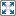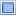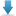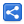# Java Regex - Basic Constructs

[Last Updated: Dec 4, 2017]

Java provides support for searching a given string against a pattern specified by the regular expression.

Followings are the `java.util.regex` classes/methods, we are going to cover in these tutorials.

• The static method `Pattern#matches` can be used to find whether the given input string matches the given regex. e.g. `Pattern.matches("xyz", "xyz")` will return true. The first argument is regex, and second is the input string.
• We can also compile the regex pattern using another static method `Pattern#compile("theRegex")`. This method returns instance of `Compile`. If a pattern is reused multiple times we should use the same compiled pattern for performance reasons.
• After getting the instance of `Pattern`, we can call `patternInstance#matcher("theInputString")` which returns instance of `Matcher`. This object is a stateful Object, meaning we can call it's method `Matcher#find` multiple times. Each time we call it and if it returns true then we found a match. At that point we can use other methods like `Matcher#start()`, `Matcher#end()` and `Matcher#group()` to find start index, end index and the string value of the match respectively. Note that `Matcher` class implements `MatchResult` interface which defines query methods to determine the results of a match against a regular expression.
• `Matcher#matches` is equivalent to static call `Pattern#matches`, but works with the underlying compiled pattern.
• The Difference between `find()` and `matches()` is that, matches() tries to match the expression against the entire string. Whereas, find() can match in a substring.
• `Matcher#replace("newString")` is also useful to replace parts with the new string which is based on underlying regex pattern.
• `Matcher#reset()`, discards all of its explicit state information and sets its append position to zero. That means next find() will start from beginning.

#### java.lang.String support of Regex

Operations provided by `String` class: `matches()`, `replaceAll(), replaceFirst()` and `split()` supports Regex. For example `String#matches` will redirect call to `Pattern#matches`

#### Example

This example is just to show how we can use Pattern/Matcher class methods.

``` String regex = "SomeRegex";
String input = "This is my input string to test SomeRegex to " +
"see how many matches we will have with SomeRegex.";

System.out.printf("using Pattern#matches: %s \n",
Pattern.matches(regex, input));

Pattern pattern = Pattern.compile(regex);
Matcher matcher = pattern.matcher(input);

while (matcher.find()) {
String matchedValue = matcher.group();
System.out.printf("Matched startIndex= %s, endIndex= %s, match: '%s'\n",
matcher.start(), matcher.end(), matchedValue);
}
```

Output:

```using Pattern#matches: false
Matched startIndex= 32, endIndex= 41, match: 'SomeRegex'
Matched startIndex= 84, endIndex= 93, match: 'SomeRegex'
```

In above example, Pattern#matches doesn't match the pattern because the method matches tries to match the pattern against entire expression. `Pattern.matches("SomeRegex", "SomeRegex")` will return true.

#### Regular Expression Basic Constructs with Examples

In the right column, we are going to give examples. We are going to put comments in the code to mention the output. In case of find() method calls, we are going to display the match along with index ranges. If there are multiple ranges, you have to call find() method multiple times unless there's no match.

Regex Construct/Terms Examples
Literals in Regex:

A literal is any character(s) we use in regular expression to search. e.g. the in Mathematics.

```Pattern.matches("in", "Linux"); //false
/* matches() tries to match the expression against the entire string. Whereas, find() can match in a substring*/
Pattern.compile("in")       .matcher("Linux")       .find();//matches:  'in' at 1-3
//'Linux'

Pattern.compile("in")       .matcher("Linux")       .replaceAll("u");//result: 'Luux'

```
.
The dot.

One dot represents exactly one character. We can put multiple dots followed by each other. We can actually put any regex construct/literal one after another and they are matched in the same sequence. Dot does not match \r or \n.
It is one of the metacharacters. A metacharacter is one or more special characters that have a special meanings to the regex engine and are not considered as literals.

```Pattern.matches(".", "a"); //true
Pattern.matches(".", " "); //true
Pattern.matches(".z", "cz"); //true
Pattern.matches(".z", "cb"); //false
Pattern.matches(".z", "9z"); //true
Pattern.matches("..e", "the"); //true
Pattern.matches("t.e", "the"); //true
Pattern.matches("...", "the"); //true
Pattern.compile("i.u")       .matcher("Linux")       .replaceAll("yn");//result: 'Lynx'

```
[ ]
Character classes:

We put multiple characters in the bracket. They are matched using 'or' logic. That means a squared bracket in an expression with match only one input character.

 [xy] x or y (can be more than two) [^xy] Any char but x or y. (^ negates if it's inside a squared bracket. It's a metacharacter.) [a-zA-Z] Any char from a to z or A to Z (range). Anything like [a-Z] or [a-9] will result in `PatternSyntaxException` [a-d[s-z]] same as [a-ds-z] (union). We can also do something like this [[a-d][s-z]]. [[a-d][^p-r]] This is equivalent to [a-os-z] [a-s&&[n-v]] Any character n to s i.e. [n-s] (intersection)
```Pattern.matches("[0-9]", "9"); //true
Pattern.matches("[[0-9][a-z]]", "t"); //true
Pattern.matches("[[0-9][^e-z]]", "s"); //false
Pattern.matches("[a-z][0-9]", "t5"); //true
Pattern.matches("[a-z&&[n-q]]", "s"); //false
Pattern.matches("[a-z&&[n-q]]", "o"); //true
Pattern.matches("[jJ][aA][vV][aA]", "jAva"); //true
Pattern.matches(".[aA][vV][aA]", "mAva"); //true
Pattern.matches("[jJ][aA].[aA]", "lAva"); //false
Pattern.matches("[A-Z][a-z].java", "My.java"); //true
Pattern.matches("[A-Z][a-z].java", "My8java"); //true
/* We have to escape '.' for it to behave as normal character and not as the metacharacter. For escaping we have to use double backslash.*/
Pattern.matches("[A-Z][a-z]\\.java", "My8java"); //false
Pattern.matches("[A-Z][a-z]\\.java", "My.java"); //true
/* We can instead put '.' inside squared brackets. In that case we don't have to escape it.*/
Pattern.matches("[A-Z][a-z][.]java", "My8java"); //false
Pattern.matches("[A-Z]", "USA"); //false
/* In next example, if called three times find() will match all three characters.*/
Pattern.compile("[A-Z]")       .matcher("USA")       .find();//matches:  'U' at 0-1, 'S' at 1-2, 'A' at 2-3
//'USA'

Pattern.matches("[1-9][1-9]-[1-9][1-9]",
"38-99"); //true
```
Predefined Character Classes:
 \d Represents exactly one digit. Equivalent to [0-9] \D Represents exactly one non-digit. Equivalent to [^0-9] \w Represents exactly one word character. Equivalent to [a-zA-Z_0-9] \W Represents exactly one non-word character. Equivalent to [^\w] \s Represents exactly one whitespace character. Equivalent to [\t\n\x0B\f\r] \S Represents exactly one non-whitespace character. Equivalent to [^\s]
```Pattern.matches("\\d", "4"); //true
Pattern.matches("\\d", "c"); //false
Pattern.matches("\\D", "a"); //true
Pattern.matches("\\W", "c"); //false
/* In following two examples both are false because white-space is not an alphabet nor a digit*/
Pattern.matches("\\w", " "); //false
Pattern.matches("\\d", " "); //false
Pattern.matches("\\s", " "); //true
Pattern.matches("\\D", " "); //true
Pattern.matches("\\W", " "); //true
Pattern.matches("\\w", "cd"); //false
/* Last one returns false because there has to be only one alphabet for one \\w */
Pattern.compile("\\w")       .matcher("cd")       .find();//matches:  'c' at 0-1, 'd' at 1-2
//'cd'

Pattern.matches("\\w\\w", "cd"); //true
Pattern.matches("[\\D]\\d", "b4"); //true
Pattern.matches("[a-z]\\s[0-9]", "a 4"); //true
Pattern.matches("\\D\\d\\w\\W", "w9s4"); //false
Pattern.matches("\\w\\W", "_@"); //true
Pattern.matches("\\W", "."); //true
Pattern.matches("\\.", "."); //true
```
Boundary Matching:
 ^ The beginning of a line. \$ The end of a line. A line ends with the character \n or \r. For example "first line\nsecond line" \b A word boundary. A word boundary can be defined as the position where a word character is followed by a non-word character and vice-versa. \B A non-word boundary. \G The end of the previous match \Z The end of the input but for the final terminator, if any \z The end of the input. \A The beginning of the input.
```Pattern.matches("^The", "The line"); //false
Pattern.compile("^The")       .matcher("The line")       .find();//matches:  'The' at 0-3
//'The line'

Pattern.compile("^The")       .matcher("This is The line")       .find();//no matches

Pattern.compile("line\$")       .matcher("The line")       .find();//matches:  'line' at 4-8
//'The line'

Pattern.compile("\\bline")       .matcher("The line")       .find();//matches:  'line' at 4-8
//'The line'

Pattern.compile("is")       .matcher("This is island")       .find();//matches:  'is' at 2-4, 'is' at 5-7, 'is' at 8-10
//'This is island'

Pattern.compile("\\bis")       .matcher("This is island")       .find();//matches:  'is' at 5-7, 'is' at 8-10
//'This is island'

Pattern.compile("\\bis\\b")       .matcher("This is island")       .find();//matches:  'is' at 5-7
//'This is island'

Pattern.compile("line")       .matcher("The inclined line")       .find();//matches:  'line' at 7-11, 'line' at 13-17
//'The inclined line'

Pattern.compile("\\bline")       .matcher("The inclined line")       .find();//matches:  'line' at 13-17
//'The inclined line'

Pattern.compile("line\\b")       .matcher("The inclined line")       .find();//matches:  'line' at 13-17
//'The inclined line'

Pattern.compile("lined\\b")       .matcher("The inclined line")       .find();//matches:  'lined' at 7-12
//'The inclined line'

Pattern.compile("\\bi")       .matcher("water is inside inland")       .find();//matches:  'i' at 6-7, 'i' at 9-10, 'i' at 16-17
//'water is inside inland'

Pattern.compile("\\bin")       .matcher("water is inside inland")       .find();//matches:  'in' at 9-11, 'in' at 16-18
//'water is inside inland'

/* Following example specifies Pattern.MULTILINE so that ^ and \$ will be used to match at the start and end of each line (otherwise match will be at the start/end of the entire string).*/
Pattern.compile("^T", Pattern.MULTILINE)       .matcher("The First line\nThe SecondLine")       .find();//matches:  'T' at 0-1, 'T' at 15-16
//'The First line\nThe SecondLine'

/* There can be \r or \r\n in the input string as line terminator*/
Pattern.compile("^a\\w", Pattern.MULTILINE)       .matcher("a an \r\napple")       .find();//matches:  'ap' at 7-9
//'a an \r\napple'

Pattern.compile("\\Aa", Pattern.MULTILINE)       .matcher("a \napple")       .find();//matches:  'a' at 0-1
//'a \napple'

Pattern.compile("\\Ga")       .matcher("aab 421aa")       .find();//matches:  'a' at 0-1, 'a' at 1-2
//'aab 421aa'

```
|
The logical Operator 'OR'

For example X|Y means Either X or Y

```Pattern.matches("a|b", "a"); //true
Pattern.compile("a|b")       .matcher("alphabet")       .replaceAll("X");//result: 'XlphXXet'

Pattern.matches("[a-d]|[x-z]", "x"); //true
Pattern.matches("[a-d][x-z]|[^*&%]", "cy%"); //false

/* This will still match. Engine will match either everything to the left or to the right of the pipe*/
Pattern.compile("Gravity|levity")       .matcher("levity Gravity Gravitlevity")       .find();//matches:  'levity' at 0-6, 'Gravity' at 7-14, 'levity' at 21-27
//'levity Gravity Gravitlevity'

Pattern.compile("Lauretta Demaria|Jannette Ballard")       .matcher("Jannette Ballard")       .find();//matches:  'Jannette Ballard' at 0-16
//'Jannette Ballard'

Pattern.compile("This is Lauretta Demaria|Jannette Ballard")       .matcher("This is Lauretta Demaria")       .find();//matches:  'This is Lauretta Demaria' at 0-24
//'This is Lauretta Demaria'

/* The complete sentence doesn't match because of the same reason mentioned above. We have to use groups (next section) for this kind of situations.*/
Pattern.compile("This is Lauretta Demaria|Jannette Ballard")       .matcher("This is Jannette Ballard")       .find();//matches:  'Jannette Ballard' at 8-24
//'This is Jannette Ballard'

```
( )
Grouping:

Grouping the expressions together is very useful if used with logical or i.e. |.
Groups can be used to capture values as well. We will cover capturing groups in advance tutorial. Here we going to give some examples in the right column to demonstrate how grouping can be used for alteration.

```Pattern.compile("This is (Lauretta Demaria|Jannette Ballard)")       .matcher("This is Jannette Ballard")       .find();//matches:  'This is Jannette Ballard' at 0-24
//'This is Jannette Ballard'

Pattern.compile("l(og|yr)ic")       .matcher("logic lyric loyric")       .find();//matches:  'logic' at 0-5, 'lyric' at 6-11
//'logic lyric loyric'

Pattern.compile("l(og|yr)ic")       .matcher("logic lyric loric")       .find();//matches:  'logic' at 0-5, 'lyric' at 6-11
//'logic lyric loric'

Pattern.matches("[a-z&&([n-q]|[u-x])]", "p"); //true
Pattern.matches("[a-z&&([n-q]|[u-x])]", "t"); //false

/* Following example shows the 12 hour time regex*/
Pattern.matches("([1-9]|1):[0-5][0-9]",
"3:59"); //true
Pattern.matches("([1-9]|1):[0-5][0-9]",
"3:70"); //false
```

# Example Project

Dependencies and Technologies Used:

• JDK 1.8
• Maven 3.0.4

 Java Regex Basic ExamplesSelect AllDownload• java-regex-examples
• src
• main
• java
• com
• logicbig
• example
• RegexExamples.java

 Share